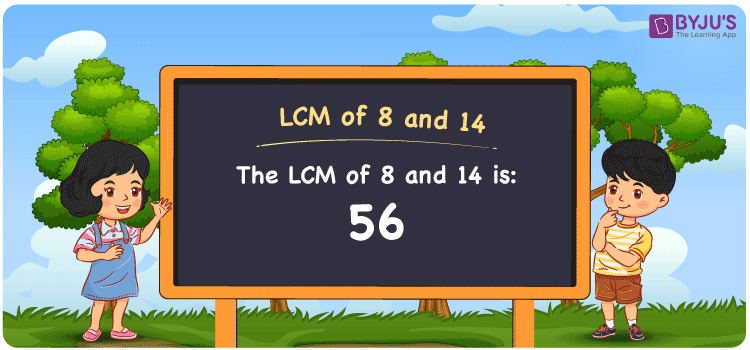Checkout JEE MAINS 2022 Question Paper Analysis : Checkout JEE MAINS 2022 Question Paper Analysis :

# LCM of 8 and 14

LCM of 8 and 14 is 56. The top-grade faculty with good knowledge of the LCM concept curated the article LCM of Two Numbers in a clear format based on the understanding abilities of students. Referring to this article in detail, students get to learn how to find the least common multiple of two numbers more effectively. Grasping the LCM concept thoroughly enables students to answer tricky questions in exams easily. Let us glance at how to find the least common multiple of 8 and 14 in a simple process in this article.

## What is LCM of 8 and 14?

The Least Common Multiple of 8 and 14 is 56.## How to Find LCM of 8 and 14?

The methods used to find the LCM of 8 and 14 are:

• Prime Factorisation
• Division method
• Listing the multiples

### LCM of 8 and 14 Using Prime Factorisation Method

By prime factorisation method, we can write 8 and 14 as the product of prime numbers, such that;

8 = 2 × 2 × 2

14 = 2 × 7

LCM (8, 14) = 2 × 2 × 2 × 7 = 56

### LCM of 8 and 14 Using Division Method

In this method, to calculate the LCM, we divide the numbers 8 and 14 by their prime factors until we get the result as one in the complete row. The product of these divisors depicts the least common multiple of 8 and 14. This can be shown as below.

 2 8 14 2 4 7 2 2 7 7 1 7 x 1 1

No more further division can be done.

Hence, LCM (8, 14) = 2 × 2 × 2 × 7 = 56

### LCM of 8 and 14 Using Listing the Multiples

Here, we list out the multiples of 8 and 14 to find their least common multiple. The following tables give the multiples of 8 and 14.

 Multiples of 8 Multiples of 14 8 14 16 28 24 42 32 56 40 70 48 84 56 98 64 112

LCM (8, 14) = 56

## Related Articles

Prime Factorisation and Division Method for LCM and HCF

Least Common Multiple (LCM)

LCM Calculator

LCM Formula

LCM with Examples

HCF and LCM

## Video Lesson on Applications of LCM## Solved Examples

Q. 1: What is the smallest number that is divisible by both 8 and 14?

Solution: 56 is the smallest number that is divisible by both 8 and 14.

Q. 2: Find the LCM of 8 and 14 using listing multiples.

Solution: First, list the multiples of 8 and 14

Multiples of 8 = 8, 16, 24, 32, 40, 48, 56, 64, 72, …….

Multiples of 14 = 14, 28, 42, 56, 70, 84, 98, 112, …..

Here the least common multiple is 56

Therefore the LCM of 8 and 14 by listing multiples is 56.

## Frequently Asked Questions on LCM of 8 and 14

### What is the LCM of 8 and 14?

The LCM of 8 and 14 is 56.

### Is the LCM of 8 and 14 the same as the HCF of 8 and 14?

No. LCM of 8 and 14 is 56 and HCF of 8 and 14 is 2.

### What are the common prime factors of 8 and 14?

2 is the only common prime factor of 8 and 14.